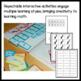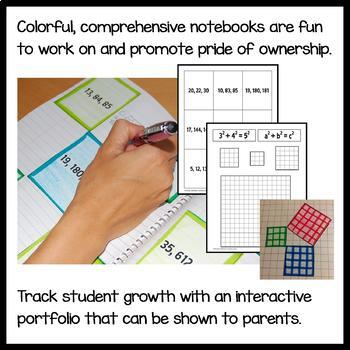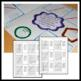# Algebra Interactive Notebook Common Core with Scaffolded NotesSubject
Resource Type
Format
Zip (28 MB|461 pages)
Standards
\$39.95
Also included in
1. Interactive Math Notebook Bundle for 6th grade, 7th grade, 8th grade, pre-algebra, algebra, and geometry - 2,657 pages! Scaffolded Notes have been added for 6th grade math, 7th grade math, 8th grade math, pre-algebra, algebra, and geometry. ANSWER KEYS ARE INCLUDED! 40 interactive printables will
\$143.82
\$239.70
Save \$95.88
• Product Description
• Standards

Algebra Interactive Notebook has 461 pages filled with hands-on activities. ANSWER KEYS ARE INCLUDED! This huge bundle has everything you need for a full year of teaching algebra. Scaffolded notes have been added which are 110 pages! Also to be included will be 40 interactive printables with answer keys to accompany your lessons. They can be used for homework, bell work, morning work, sub work or for reinforcement. Great for Distance Learning!

This Algebra interactive notebook is completely hands-on and interactive. Each chapter in this interactive math journal includes a divider for the standard that is covered in the chapter, a hands-on activity for students to put in their math notebooks, and at least one page that you can use as an assessment or as a worksheet for additional practice. In addition, each chapter has a page of cut-outs that your students can color, cut out, and paste into their math notebooks. There are also pictures of pages from this notebook to give you ideas about how to set up your Common Core math notebooks.

Also included in this product are 110 pages of scaffolded notes or guided notes and activities. Students will be more engaged in your lessons when they have these notes to help guide their learning. Answer keys are included.

All Common Core State Standards for Algebra 1 (often studied in ninth grade/freshman year) are covered in this book.

Topics covered:

Interpret Expressions

Rewriting Expressions

Equivalent Forms of Expressions

Understanding Functions

Understanding Polynomials

The Remainder Theorem

Identifying Zeros of Polynomials

Prove and Use Polynomial Identities

Binomial Theorem

Rewriting Rational Expressions

Understand Rational Expressions

Create Equations and Inequalities

Equations Representing Variables

Representing Constraints

Rearranging Formulas

Explaining Steps in Equations

Linear Equations

Systems of Equations

More Linear Equations

Single Matrix Equation

Inverse of a Matrix

Graph of an Equation

X-Coordinates

Graphing Linear Inequalities

***

***

If you are interested in this book for kindergarten, click here .

If you are interested in this book for Pre-Algebra, click here .

If you are interested in this book for Geometry, click here .

If you are interested in this book for Algebra 2, click here .

All graphics are originals and created by myself.

Thanks for visiting my store,

Yvonne Crawford

to see state-specific standards (only available in the US).
Graph the solutions to a linear inequality in two variables as a half-plane (excluding the boundary in the case of a strict inequality), and graph the solution set to a system of linear inequalities in two variables as the intersection of the corresponding half-planes.
Explain why the 𝘹-coordinates of the points where the graphs of the equations 𝘺 = 𝘧(𝘹) and 𝘺 = 𝑔(𝘹) intersect are the solutions of the equation 𝘧(𝘹) = 𝑔(𝘹); find the solutions approximately, e.g., using technology to graph the functions, make tables of values, or find successive approximations. Include cases where 𝘧(𝘹) and/or 𝑔(𝘹) are linear, polynomial, rational, absolute value, exponential, and logarithmic functions.
Understand that the graph of an equation in two variables is the set of all its solutions plotted in the coordinate plane, often forming a curve (which could be a line).
Find the inverse of a matrix if it exists and use it to solve systems of linear equations (using technology for matrices of dimension 3 × 3 or greater).
Represent a system of linear equations as a single matrix equation in a vector variable.
Total Pages
461 pages
Included
Teaching Duration
1 Year
Report this Resource to TpT
Reported resources will be reviewed by our team. Report this resource to let us know if this resource violates TpT’s content guidelines.
• Ratings & Reviews
• Q & A

Teachers Pay Teachers is an online marketplace where teachers buy and sell original educational materials.